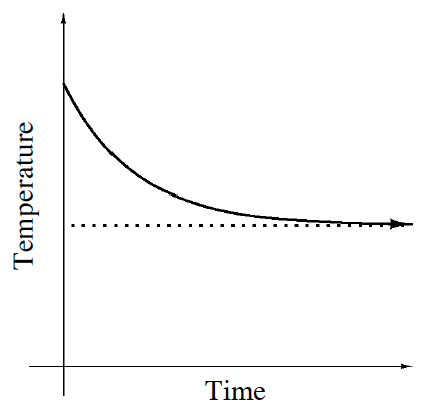Home > INT1 > Chapter 11 > Lesson 11.1.1 > Problem11-9

11-9.

The graph below represents the temperature of a cup of hot water that is left on a kitchen table.

1. What does the horizontal line (asymptote) represent?

The line appears to be the lowest temperature that the water can reach.
In a room, the lowest temperature that something can reach is the temperature of the room.

2. How would the graph be different if the cup of hot water was left outside during winter instead?

If a cup were outside in the cold, the lowest temperature that the liquid could reach would still be the temperature of its surroundings.

The asymptote would be lower, but still parallel to the $x$-axis.
If the temperature outside was below zero, the asymptote would be below the $x$-axis.